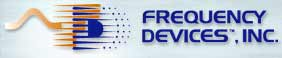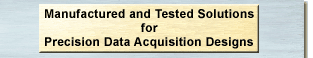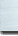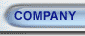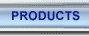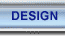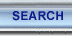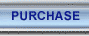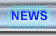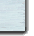July 2003

# GLOSSARY OF TERMS

ADC: An Analog to Digital converter, a device that converts a continuous analog signal into a sampled numerical code representing the original signal.

Aliasing: The result of sampling an analog frequency signal at a rate below that required by the Nyquist theorem of 2 x Fs. Under-sampling translates high frequency components into the pass-band, producing unwanted signal components and unpredictable results.

Amplitude Filters: Designed for the best amplitude response for a given situation, i.e. Zero ripple in the amplitude response pass-band.

Amplitude Response: The ratio of the output amplitude to the input amplitude versus frequency.  Amplitude response is usually plotted on a log/log scale

Anti-alias Filter: The pre-filtering of an analog signal, before it is digitized or sampled, to remove or substantially attenuate the undesired aliasing components.

Arithmetic Center Frequency: Fo=(Fc1 + Fc2)/2, this formula is not correct for specifying BAND-PASS or BAND-REJECT filters, where the formula for the GEOMETRIC CENTER is used instead.

Attenuation: The reduction in signal amplitude imposed by a filter or other electronics circuit upon an input signal.

Attenuation Floor: The maximum attenuation a filter needs to provide in the STOP-BAND.

Band-Pass Filter: A filter, which passes only signal frequencies within a specified, continuous frequency band, and predictably attenuates frequencies above and below the continuous band.

Band-Reject Filter: Also known as a "notch" filter, this filter rejects signal frequencies within a specified band, while passing out-of-band signals.

Bandwidth (BW): BW = Fc1 - Fc2, the range of frequencies between the lower and upper CORNER FREQUENCIES of a BAND-PASS FILTER or BAND-REJECT FILTER.  Usually defined at the frequencies where the response curve passes through the -3 dB points.

Center Frequency, Fo: Defined for BAND-PASS and BAND-REJECT filters as the frequency at the GEOMETRIC MEAN of two CORNER FREQUENCIES (usually -3 dB).

Clock-Feed-Through: An extraneous signal generated by the clock in switched capacitor filters.

Corner Frequency, Fc: The transition frequency range between the PASS-BAND and STOP-BAND.  For Butterworth and Bessel type filters this would be the frequency where the signal is –3.01 dB with reference to the PASS-BAND amplitude.  For other transfer functions such as Cauer elliptics, the corner is defined mathematically as ripple-frequency (Fr).

DAC: A Digital to analog converter is an electronic device, which takes input information in digital form and converts it to an analog output containing the same information.

DC-Offset: A constant voltage added to an input signal.  DC-offset can vary with time, temperature and/or changes in corner-frequency settings.

Decibel (dB): A handy way of expressing the ratio of one signal to another.  dB is calculated by 10 * log10(P2/P1), where P2, P1 are power levels.  Voltage ratios in dB are calculated by 20 * log10(V2/V1).

Decade: A 10:1 increase or decrease of a variable, usually frequency.  A 20 dB/decade gain roll-off defines a gain change of 20 dB for each 10-fold increase or decrease in frequency.  Note that 6dB/octave is equivalent to 20 dB/decade.

Delay: The time difference in the starting points of two signals, both having the same frequency and measured in seconds.

Digital Filter: Software configured filters that are used to condition digitized signals utilizing DSP's (Digital Signal Processor's) or hardware structures such as certain types of Gate Arrays.  These structures can be configured to mathematically manipulate digital data to provide the equivalent of analog filters or to produce filters not realizable in the analog world.

Distortion: Non-linearity present or imposed upon a signal by the signal generator and/or the signal processing.  Distortion is undesirable if high precision signal processing is required.

DSP: Digital Signal Processors are types of small programmable processors capable of directly manipulating digital data in a variety of ways to signal condition or analyze incoming data.  Some applications for DSP's are Cell-phones, CD & MP-3 players, and digital filters.

Electronic Filter: Electronic devices that implement filtering techniques to separate desirable signals from undesirable signals for a given application. These devices may also include capabilities to condition the signals by amplification or other processing.  Note: depending upon requirements, filters may be created using analog or digital techniques.

Equiripple Technique: A digital FIR filter design alternative to the window technique that allows designers to achieve a desired frequency response with the fewest number of coefficients.

FIR filter: A Finite Impulse Response Digital Filter, usually consisting only of Zeros (no Poles), and generally implemented by a fixed point DSP processor to produce at low cost, Equiripple digital filters.

Frequency: F =1/T, the repetition rate of a sine wave or other periodic waveform in cycles/second or Hertz (Hz).

Gain: The ratio of output signal amplitude to input signal amplitude. (Vo/Vi).

Gain in dB: The difference of output signal amplitude to input signal amplitude expressed in decibels.

Geometric Center Frequency (Fo): Fo = Square root of (Fc1*Fc2).  Used to specify Fo for BAND-PASS or BAND-REJECT filters.

Group Delay: The instantaneous rate of change of phase with respect to frequency of a filter, usually shown in a phase versus frequency plot.

Harmonic: A signal whose frequency of interest is an integer multiple of the fundamental frequency, i.e. F = fundamental, 2 x F = 2nd harmonic, 3 x F = 3rd harmonic, etc...

High-Pass Filter: This filter passes all frequencies above and rejects all frequencies below a specified CORNER FREQUENCY.

IIR filter: Infinite Impulse Response Digital Filter consists of the equivalent of both poles and zeros, generally implemented with a floating point DSP processor and capable of mathematically emulating most analog filters.

Infinite Recursive: Algorithms that utilize previously calculated values in future calculations.  Related to floating point DSP's and IIR digital filter design.

Low-Pass Filter: This filter passes all frequencies below and rejects all frequencies above a specified CORNER FREQUENCY.

Matched Response Filters: A collection of filters specifically designed with gain and phase matched responses for multi-channel systems where channel-to-channel differences must be minimized.

Monotonic Roll Off: Constantly increasing attenuation with frequency.

Non-Recursive: A repetitive delay-and-add format utilized in fixed-point math.  The finite number of "n" delay taps on a delay line and "n" computation coefficients needed to compute a digital filter algorithm.  Related to fixed-point DSP's and FIR digital filter design.

Normalized Response: The ratio between a fixed reference and a variable value, both having the same fundamental physical units.  A convenient way of comparing the response of a family of filters by plotting gain versus the ratio Fc/F, allowing the user to scale the curves up or down to meet his requirements.

Noise: Unwanted signals that distort or affect the signals of interest, and one of the reasons filters are used. Noise may come from external sources (Radiated noise such as EMI) be generated by system components (Amplifiers, resistors or clocks) or just may be an undesirable part of the signal itself.

Nyquist Frequency: To accurately represent an analog signal with samples, requires that the original signal's highest frequency be less than ½ the sampling frequency or alternatively, the Nyquist theorem sampling rate must be at least twice the analog signal frequency.

Octave: A doubling or halving, usually applied to frequency.  A GAIN ROLL-OFF RATE of 6dB/octave defines a change of 6 dB for each doubling or halving of frequency.  Note that 20 dB/decade is equivalent to 6 dB/octave.

Order: The ORDER of a filter is the number POLES in the transfer function.  The higher the ORDER, the steeper the filter's transition band slope.  (No less than –6dB/octave per pole.)

Pass-Band Ripple: The specified amount (typically in dB) of permissible amplitude variation in the PASS BAND.  This term is usually associated with Chebychev and Cauer elliptic filters.

Pass-Band: Range of frequencies throughout which a filter passes signals with no change.

Period: T = 1/f in seconds.  The time interval, which sine waves (or other periodic waveforms) repeats.

Phase: The angular measure of the difference in that starting point of two sine waves or periodic signals.  Measured in degrees or radians.

Phase Filters: These filters are designed to achieve a desired phase response, i.e. Preserve linear phase with frequency throughout the filter amplitude pass-band in order to preserve a transient waveform.

Phase Response: Is the phase difference (in degrees) between the filter input and output signals. Phase will typically shift with changes in frequency.  See GROUP DELAY for more information.

Poles: Values of complex frequency, which make the transfer function infinite.  Factors in the denominator of the transfer function polynomial.

Q (Quality Factor): For BAND-PASS and BAND-REJECT filters Q = Fo/BW.  Note that Fo is the GEOMETRIC CENTER.

Ripple Frequency: A variation in amplitude within the pass-band of amplitude filters.

Roll-Off Rate: The slope of the filter curve approaching -6 dB/octave/pole far away from the CORNER FREQUENCY.  The ROLL-OFF RATE near the CORNER FREQUENCY depends on the ORDER and the filter TYPE.

Settling Time: Mathematically related to the filter transfer function, settling time (See Delay) represents the time required for a filter transfer function to stabilize following introduction of a signal.  General guide-line, the more the filter approaches a brick-wall approximation, the longer it will take to settle.

Shape Factor: The ratio between two frequencies - the frequency at a specified attenuation on the roll-off curve to the CORNER FREQUENCY at its specified attenuation, usually 3 dB or the mathematical corner frequency depending on the filter type.

Step Response: The response of a filter to a sudden change in voltage amplitude at the filter (See Amplitude Filters) input can result in overshoot (ringing).

Stop-Band: Range of frequencies throughout which a filter attenuates signals by a defined amount.

Stop-Band Attenuation: Specifies the minimum amount of ATTENUATION a filter will exhibit at a designated frequency or range of frequencies, which lie outside the pass band.

Total Harmonic Distortion (THD): A specification used as a single number representation of the distortion present in the output of an active circuit.  It is the RMS sum of the individual harmonic distortions (i.e. 2nd, 3rd, --- etc.) that are created by the non-linearity of the active and passive components in the circuit when it is driven by a pure sinusoidal input at a given amplitude and frequency.

Transition band: The non-ideal range of frequencies contained in the pass band usually bounded between the corner frequency Fc and the attenuation floor defined by the transfer function.

Type Filter: Butterworth, Bessel, Chebychev, Cauer elliptic, constant delay, FIR, IIR or other special response characteristic.

Window Technique: A design technique for digital FIR filters that utilizes well-known frequency domain transition functions.

Zeros: Values that make the transfer function zero.  Factor in the numerator of the transfer function polynomial.

 Frequency Devices, Inc. Your engineering partner for signal conditioning 927 Fosse Rd Ottawa, IL 61350 e-mail: sales@freqdev.com Phone: (815) 434-7800